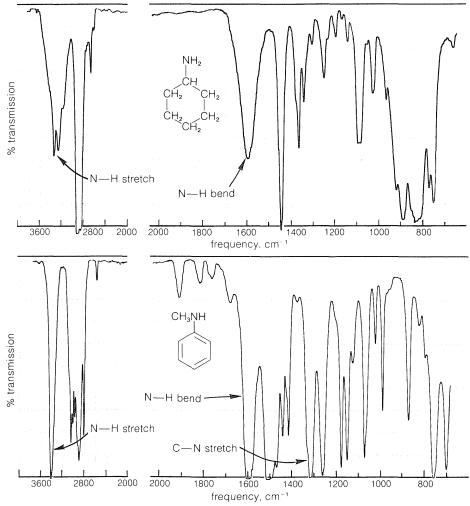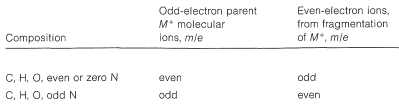# 23.5: Spectroscopic Properties of Amines

$$\newcommand{\vecs}{\overset { \rightharpoonup} {\mathbf{#1}} }$$ $$\newcommand{\vecd}{\overset{-\!-\!\rightharpoonup}{\vphantom{a}\smash {#1}}}$$$$\newcommand{\id}{\mathrm{id}}$$ $$\newcommand{\Span}{\mathrm{span}}$$ $$\newcommand{\kernel}{\mathrm{null}\,}$$ $$\newcommand{\range}{\mathrm{range}\,}$$ $$\newcommand{\RealPart}{\mathrm{Re}}$$ $$\newcommand{\ImaginaryPart}{\mathrm{Im}}$$ $$\newcommand{\Argument}{\mathrm{Arg}}$$ $$\newcommand{\norm}{\| #1 \|}$$ $$\newcommand{\inner}{\langle #1, #2 \rangle}$$ $$\newcommand{\Span}{\mathrm{span}}$$ $$\newcommand{\id}{\mathrm{id}}$$ $$\newcommand{\Span}{\mathrm{span}}$$ $$\newcommand{\kernel}{\mathrm{null}\,}$$ $$\newcommand{\range}{\mathrm{range}\,}$$ $$\newcommand{\RealPart}{\mathrm{Re}}$$ $$\newcommand{\ImaginaryPart}{\mathrm{Im}}$$ $$\newcommand{\Argument}{\mathrm{Arg}}$$ $$\newcommand{\norm}{\| #1 \|}$$ $$\newcommand{\inner}{\langle #1, #2 \rangle}$$ $$\newcommand{\Span}{\mathrm{span}}$$$$\newcommand{\AA}{\unicode[.8,0]{x212B}}$$

## Infrared and Ultraviolet Spectra

A characteristic feature of the infrared spectra of primary and secondary amines is the moderately weak absorption at $$3500 \: \text{cm}^{-1}$$ to $$3300 \: \text{cm}^{-1}$$, which corresponds to $$\ce{N-H}$$ stretching vibrations. Primary amines have two such bands in this region, whereas secondary amines generally show only one band. Absorption is shifted to lower frequencies by hydrogen bonding, but because $$\ce{NH} \cdots \colon \ce{N}$$ bonding is weaker than $$\ce{OH} \cdots \colon \ce{O}$$ bonding, the shift is not as great and the bands are not as intense as are the absorption bands of hydrogen-bonded $$\ce{O-H}$$ groups (see Table 9-2). Bands corresponding to $$\ce{N-H}$$ bending vibrations are observed around $$1600 \: \text{cm}^{-1}$$. Absorptions corresponding to $$\ce{C-N}$$ vibrations are less easily identifiable, except in the case of arenamines, which absorb fairly strongly near $$1300 \: \text{cm}^{-1}$$. Spectra that illustrate these effects are shown in Figure 23-4.Figure 23-4: Infrared spectra of cyclohexanamine and $$\ce{N}$$-methylbenzenamine ($$\ce{N}$$-methylaniline).

The ultraviolet absorptions of simple saturated amines occur at rather short wavelengths $$\left( \sim 220 \: \text{nm} \right)$$ and are not particularly useful for identification. These are $$n \rightarrow \sigma^*$$ transitions that correspond to the antibonding $$\sigma$$ orbital of a $$\ce{C-N}$$ bond.

## NMR Spectra

The proton nmr spectra of amines show characteristic absorptions for $$\ce{H-C-N}$$ protons around $$2.7 \: \text{ppm}$$. The positions of the resonances of $$\ce{N-H}$$ protons show considerable variability as the result of differences in degree of hydrogen bonding (Section 9-10E). Sometimes the $$\ce{N-H}$$ resonance has nearly the same chemical shift as the resonances of $$\ce{CH_3-C}$$ protons (as with $$\ce{N}$$-ethylethanamine, Figure 23-5).Figure 23-5: Nmr spectrum of $$\ce{N}$$-ethylethanamine (Diethylamine) at $$60 \: \text{MHz}$$ relative to TMS at $$0 \: \text{ppm}$$. Rapid exchange of the $$\ce{N-H}$$ protons between different amine molecules causes the $$\ce{N-H}$$ resonance to be a single peak (Section 9-10I).

A further complication associated with $$\ce{N-H}$$ and $$\ce{H-C-N}$$ resonances is their variable chemical shift and line width in the presence of acidic substances because of a chemical exchange process of the type illustrated in Equation 23-1:Depending on the rate at which the proton transfers of Equation 23-1 occur and the concentrations of the reactants, the chemical shift of the $$\ce{N-H}$$ proton will come between that of pure $$\ce{(CH_3)_2NH}$$ and pure $$\ce{HA}$$. Except at high acid concentrations, this exchange eliminates any observable coupling between the $$\ce{N-H}$$ proton and the $$\ce{N}$$-methyl protons $$\left( \ce{H-C-N-H} \right)$$; see Section 9-10I.

In Section 9-10L, we discussed $$\ce{^{13}C}$$ nmr and its many applications to structural problems. The nmr of $$\ce{^{15}N}$$ nuclei has similar possibilities but, because $$\ce{^{15}N}$$ is only $$0.37\%$$ of natural nitrogen and has an even smaller nuclear magnetic moment than $$\ce{^{13}C}$$, it is very difficult to detect $$\ce{^{15}N}$$ resonances at the natural abundance level.$$^2$$ Indeed, natural $$\ce{^{15}N}$$ has to be observed for about a $$6 \times 10^{10}$$ longer time than protons to achieve the same signal-to-noise ratio! Despite this difficulty, natural-abundance $$\ce{^{15}N}$$ spectra can be obtained for many compounds (even enzymes) and, in some cases, provide very useful chemical information (see Figure 24-4).

## Mass Spectra of Amines

The most prominent cleavage of the parent molecular ion $$\ce{M^+}$$ derived from amines occurs at the $$\ce{C}_\beta$$-$$\ce{C}_\alpha$$ bond to give an imminium ion which, for ethanamine, has $$m/e = 30$$:It is helpful in identifying the molecular ion of an organonitrogen compound to remember that the $$m/e$$ value of $$\ce{M^+}$$ will be an uneven number if the ion contains one or another odd number of nitrogen atoms. Thus ethanamine, $$\ce{C_2H_7N}$$, gives an $$\ce{M^+}$$ of $$m/e = 45$$. For all other elemental compositions of $$\ce{C}$$, $$\ce{H}$$, $$\ce{O}$$, or with an even number of nitrogens, the molecular ion will have an even $$m/e$$ value.

The cleavage reaction of Equation 23-2 reveals other useful generalizations. Whatever its source, a parent molecular ion, $$\ce{M^+}$$, has one unpaired electron and is properly described as an odd-electron ion (a radical cation). When a parent molecular ion fragments, it does so homolytically, as shown in Equation 23-2, and produces a radical and an ion in which the electrons are paired - an even-electron ion. The $$m/e$$ value of an even-electron ion is an even number for any elemental composition of $$\ce{C}$$, $$\ce{H}$$, $$\ce{O}$$ in combination with an odd number of nitrogens. These generalizations are summarized in Table 23-2 and can be useful in the interpretation of mass spectra.$$^2$$The abundant nitrogen nucleus, $$\ce{^{14}N}$$, has a magnetic moment but generally gives very poor nmr spectra with very broad lines. The reason is the $$\ce{^{14}N}$$ usually "relaxes" rapidly, which means that its nuclear magnetic states have short lifetimes (see Section 27-1).